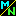Navigation Panel:(These buttons explained below)# Number Systems with Different Kinds of Numbers

In mathematics, the phrase number system refers to any collection of objects that has the following properties:

• there is a rule for how to add two objects together,

• there is a rule for how to multiply two objects together,

• the rules for addition and multiplication satisfy the familiar properties of arithmetic, such as
• commutativity (order doesn't matter),
• associativity (in a sum of three of more terms, it doesn't matter which two you add first, and likewise for products), and
• distributivity (a(b+c) = ab + ac), and

• all objects have corresponding negatives (from which we can define subtraction as the addition of one object to the negative of another), and some objects have reciprocals (from which we can define division as the multiplication of one object by the reciprocal of another). The properties of subtraction and division follow automatically from the corresponding properties of addition and multiplication, so we need not mention them explicitly.

• In some cases we might consider two objects in the collection to be equal even if they are not the same object; for instance, the fractions 1/2 and 2/4 are equal, even though they are different pairs of numbers. When this is the case, we also need, in addition to the properties above, a precise definition of when two objects are equal.

Roughly speaking, any collection of objects that satisfies these properties is, by definition, a number system.

Note: this is only a rough statement. If you wanted to say things more accurately, you'd have to take into account the fact that

• strictly speaking, some of these properties need to be stated a little more precisely than I stated them;
• the phrase "number system" is not often used; instead, one uses more fancy language such as "field" or "commutative ring"; and
• usually the rules for addition and multiplication satisfy various other properties besides the ones I have listed, and one uses terminology that precisely expresses which of these properties are present.
However, the rough statement is enough for our purposes.

### Examples of Number Systems

The most common number systems are the following:
• The Natural Numbers. These are the counting numbers 1, 2, 3, . . . that are possible answers to the question "how many?" They are abstract concepts that describe sizes of sets.

• The Integers. These are abstract concepts that describe, not sizes of sets, but the relative sizes of two sets. They are the possible answers to the question "how many more does A have than B has?" They include both positive numbers (meaning A has more than B) and negative numbers (meaning B has more than A).

• The Rational Numbers. These are abstract concepts that describe ratios of sizes of sets. They do not model sizes of sets the way that natural numbers do. If you say "I ate 3/4 of a pie", you are not saying that the set of things you ate had 3/4 elements. Instead, you are expressing a ratio of two integer quantities: 3, the number of pie-quarters that you ate, and 4, the number of pie-quarters that make up a whole pie.

• The Real Numbers. These are abstract concepts that describe measurements of continuous quantities, such as length, weight, quantity of fluid, etc. (Don't let the word "real" fool you; the real numbers are no more "real" in the ordinary English sense of the word than are any other kind of numbers.)

• The Complex Numbers. These are pairs of real numbers, with pairs of the form (x,0) behaving the same as ordinary real numbers x, but with other pairs (whose second entry is non-zero) behaving differently. The rule for multiplication is
(a, b) times (c, d) = (ac-bd, ad+bc)
which means that the pair (0,1), when squared, gives (-1,0) which in this context is considered to be the same as the real number -1 (since a complex number of the form (x,0) is indistinguishable by its arithmetic properties from the real number x, we can consider it to be describing the same underlying concept, much as we consider a fraction of the form x/1 to be describing the same underlying concept as the integer x).

Thus, the complex numbers are a number system in which there is an object whose square is -1. This object is denoted by i, and the pair (a,b) is written as "a + bi". When a=0, such a number is called an "imaginary number".

Complex numbers are extremely important mathematically. They have less direct relevance to real-world situations, since they aren't measurements of single quantities. However, they relate directly to a few real-world situations (such as the strength of an electromagnetic field), and they relate indirectly to many more since many properties of real numbers can be more easily understood in the larger context of complex numbers.

Navigation Panel: